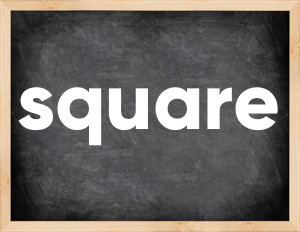# Square past tenseThe English verb 'square' is pronounced as [skwɛː].
Related to: regular verbs.
3 forms of verb square: Infinitive (square), Past Simple - (squared), Past Participle - (squared).

## Here are the past tense forms of the verb square

👉 Forms of verb square in future and past simple and past participle.
❓ What is the past tense of square.

## Square: Past, Present, and Participle Forms

Base Form Past Simple Past Participle
square [skwɛː]

squared [skweəd]

squared [skweəd]

## What are the 2nd and 3rd forms of the verb square?

🎓 What are the past simple, future simple, present perfect, past perfect, and future perfect forms of the base form (infinitive) 'square'?

### Learn the three forms of the English verb 'square'

• the first form (V1) is 'square' used in present simple and future simple tenses.
• the second form (V2) is 'squared' used in past simple tense.
• the third form (V3) is 'squared' used in present perfect and past perfect tenses.

## What are the past tense and past participle of square?

The past tense and past participle of square are: square in past simple is squared, and past participle is squared.

### What is the past tense of square?

The past tense of the verb "square" is "squared", and the past participle is "squared".

### Verb Tenses

Past simple — square in past simple squared (V2).
Future simple — square in future simple is square (will + V1).
Present Perfect — square in present perfect tense is squared (have/has + V3).
Past Perfect — square in past perfect tense is squared (had + V3).

### square regular or irregular verb?

👉 Is 'square' a regular or irregular verb? The verb 'square' is regular verb.

## Examples of Verb square in Sentences

•   She was using the id to square the debt. (Past Simple)
•   He squared any three-digit numbers (Past Simple)
•   Can you square this number? (Present Simple)
•   I'll square up with you some day (Future Simple)
•   He squared the matter with her (Past Simple)
•   They have squared the police  (Present Perfect)
•   Her actions do not always square with her promises (Present Simple)
•   His statement fails to square with the facts (Present Simple)
•   He squares other people by his own standards (Present Simple)
•   You need to square your creditors (Present Simple)

Along with square, words are popular master and focus.

Verbs by letter: , , , , , , , , , , , , , , , , , , , , , , , , .